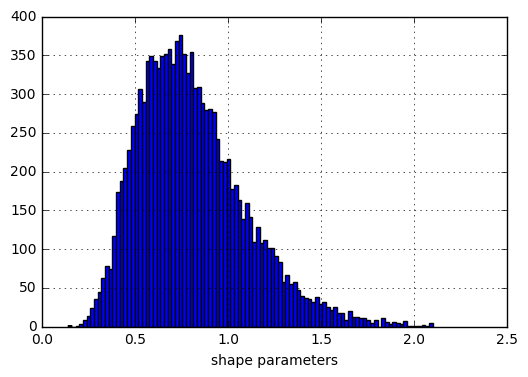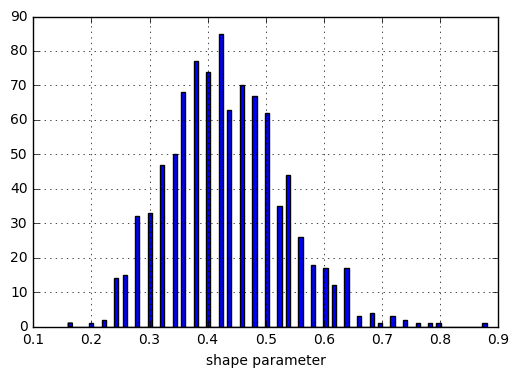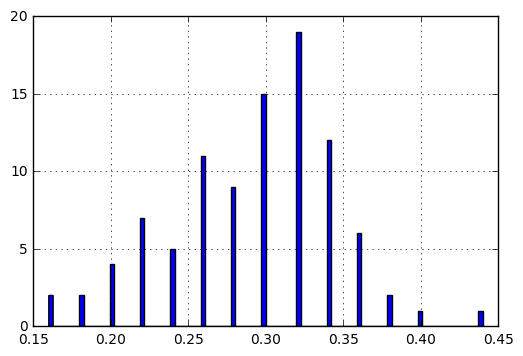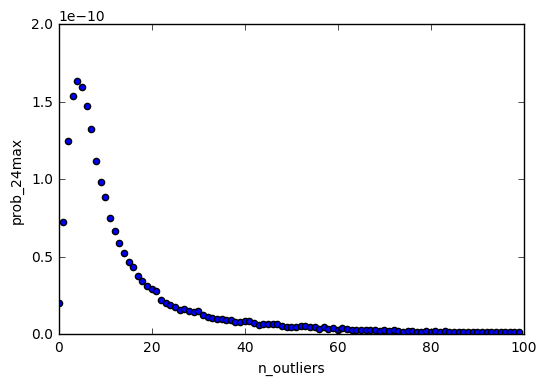# NaClhv

Theology, philosophy, math, science, and random other things

2017-01-09

## Bayesian evaluation for the likelihood of Christ's resurrection (Part 39)

This is a jupyter notebook. It contains the python code which generates the relationship between the number of "outliers" (as previously defined) and the probability of naturalistically generating a Jesus-level resurrection report.

resurrection_calculation

First, we import some modules:

In :
%matplotlib inline
import numpy as np
import pandas as pd
from scipy.stats import genpareto


Next, we write the function to simulating getting the maximum value out of n samples from a given distribution:

In :
def max_out_of_n_from_dist(dist, out_of_n=1e9):
manageable_n = 100000
if out_of_n <= manageable_n:
return dist.rvs(out_of_n).max()
else:
top_percentiles = \
np.random.rand(manageable_n) * manageable_n / out_of_n
return dist.isf(top_percentiles).max()


Next, we consider generalized Pareto distributions with the shape parameters between 0.02 to 2.1, in increasing intervals of 0.02. That is, we consider shape paramters of 0.02, 0.04, 0.06 ... 2.1.

We then simulate getting the maximum value out of 1e9 samples drawn from these distributions. We next calculate how many "outliers" would exist given that maximum value and that distribution. Lastly, we calculate the probability of drawing a sample whose value is 24 times greater than the maximum value. This is the probability of "naturally" generating a Jesus-level resurrection report for that distribution.

We repeat this 10000 times for each of the 105 shape parameters between 0.02 and 2.1, and put it all in a table. The result is a table with 1050000 rows, whose columns are the shape parameter, the number of outliers, and the probability of drawing a sample 24 times greater than the maximum.

The following code gives us this results table.

In :
sample_size = int(1e9)
genpareto_shapes = np.linspace(0.02, 2.1, 105)
shape_params = []
prob_24max = []
n_outliers_estimation = []

for shape_param in genpareto_shapes:
dist = genpareto(shape_param, scale=1, loc=0)
for i in range(10000):
shape_params.append(shape_param)
max_val = max_out_of_n_from_dist(dist, sample_size)
prob_24max.append(dist.sf(max_val * 24))
p_outlier = (dist.sf(max_val * 0.2) - dist.sf(max_val)) \
/ dist.cdf(max_val)
n_outliers_estimation.append(
int(round(p_outlier * sample_size)))

genpareto_results_df = pd.DataFrame({
"shape_params":shape_params,
"prob_24max":prob_24max,
"n_outliers":n_outliers_estimation,
})

#save to .csv, as generating this takes a while
genpareto_results_df.to_csv(
"genpareto_results_df.csv", encoding="utf-8")


Let's load up the results and see the first few rows:

In :
genpareto_results_df = pd.read_csv(
"genpareto_results_df.csv", encoding="utf-8"
).drop("Unnamed: 0", 1)

In :
print genpareto_results_df.shape

(1050000, 3)

Out:
n_outliers prob_24max shape_params
0 6579077 1.547543e-57 0.02
1 7069940 3.123026e-57 0.02
2 6141769 7.974702e-58 0.02
3 6608791 1.616688e-57 0.02
4 3459940 4.204358e-60 0.02

So, let's say that in reality, there are only 10 "outliers". Now, this does not narrow down the possibilities to a single shape parameter. Just due to chance, you can get 10 "outliers" from a shape parameter of 0.5, and also from a shape parameter of 1.5. However, the 10 "outliers" does narrow things down enough to give us a distribution over shape parameters. This is an improvement over our prior knowledge about the shape parameters, which was that we had no idea what it might be.

How could we get this posterior distribution of the shape parameters? All we need to do is to take the subset of the results table where the number of outliers is exactly 10, and look at the shape parameters. This satisfies Bayes' theorem, and gives us a posterior distribution that looks like this:

In :
genpareto_results_df[genpareto_results_df["n_outliers"] == 10]\
["shape_params"].hist(bins=99).set_xlabel("shape parameters")

Out:
<matplotlib.text.Text at 0x12e4bb38>Let's continue with the same reasoning. The probability of generating a Jesus-level resurrection report, averaged over the shape parameters in the distribution above, would simply be the average of that probability over the subset of the results table where the number of outliers is exactly 10:

In :
genpareto_results_df[genpareto_results_df["n_outliers"] == 10]\
["prob_24max"].mean()

Out:
8.840657596227429e-11

Note that, for the distribution over shape parameters, we run into the upper limit of 2.1. Meaning that the actual distribution extends to higher values of the shape parameter, and the probability of achieving a Jesus-level resurrection report would actually be higher.

Of course, there's almost certainly more than 10 "outliers" in world history. So this will not matter for our actual calculation. But it does show us that we have to be careful about bumping into the edges of our range of shape parameters. We also have to worry about the decay rate of the distribution over shape parameters, as it goes off to the right. Thankfully, it turns out that it decays quickly enough that we can simply ignore it after a certain point. The probability density beyond a shape parameter of 2.1 is negligible once we go a little bit beyond 10 "outliers". Demonstrating this is left as an exercise for the reader.

So, what if there are more outliers, like 50? That would make for the following posterior distribution over shape parameters:

In :
genpareto_results_df[genpareto_results_df["n_outliers"] == 50]\
["shape_params"].hist(bins=100).set_xlabel("shape parameter")

Out:
<matplotlib.text.Text at 0xc03cba8>And the probablity of generating a Jesus-level resurrection report would be:

In :
genpareto_results_df[genpareto_results_df["n_outliers"] == 50]\
["prob_24max"].mean()

Out:
4.428207171511614e-12

What if there are 250 outliers? Then the distribution over shape parameters looks like this:

In :
genpareto_results_df[genpareto_results_df["n_outliers"] == 250]\
["shape_params"].hist(bins=100)

Out:
<matplotlib.axes._subplots.AxesSubplot at 0x10e1c780>And the probablity of generating a Jesus-level resurrection report would be:

In :
genpareto_results_df[genpareto_results_df["n_outliers"] == 250]\
["prob_24max"].mean()

Out:
1.5911343547015349e-13

We can clearly see that the number of "outliers" controls the probability of generating a Jesus-level resurrection report. Here is how the two quantities are related:

In :
outliers_p24max = genpareto_results_df[
genpareto_results_df["n_outliers"] < 100
].groupby("n_outliers")["prob_24max"].mean()

outliers_p24max.reset_index().plot(
kind="scatter", x="n_outliers", y="prob_24max",
xlim=(0,100), ylim=(0, 2e-10)
)

Out:
<matplotlib.axes._subplots.AxesSubplot at 0xd5254a8>The abnormal values around n_outliers < 5 is due to the "shape parameter exceeding 2.1" problem mentioned earlier. It quickly becomes a non-issue as the number of outliers increases.

Looking at the rest of the graph, we see that the probability of generating a Jesus-level resurrection report drops as the number of "outliers" increases. Having MORE non-Christian resurrection reports (that is, having more "outliers") makes the skeptic LESS able to explain Jesus's resurrection, and therefore makes it MORE likely - exactly as we said before.

So, the question now just comes down to this: how many "outliers" can we find in world history? Recall that anyone with a "some people say..." level of evidence for their resurrection counts as an outlier. The more such people we can find, the more firmly Christ's resurrection is established. Can we find enough such people to overcome the low prior probability against a resurrection?

We'll get to that in the next post.

You may next want to read:
Basic Bayesian reasoning: a better way to think (Part 1)
The role of evidence in the Christian faith

### 2 comments on “Bayesian evaluation for the likelihood of Christ's resurrection (Part 39)”

1.Anonymous says:

This has been an excellent series and I look forward to having all put in book form. It's one of those books that are long overdue. I leaned a lot form it and from the comments by Professor Aron Wall in his blog.

TY

2.naclhv says:

Thank you! I do think that the major arguments in this series are new and solid, and definitely worth getting into a book form - but we'll see!